The Graph Toolbox contains functions to generate complex statistical graphs very quickly. The idea is that the user should only have to pass the raw data, and the graph function calculates the to-be-plotted values, including error bars, from the data. Variable option provide the flexibility of the style of plots and the formatting. To not have to redo the styles, there are default formatting "styles" and the possibility to store a formatting option in a structure.

Let's assume we have a dataset from a virtual experiment, in which N=20 subjects, either patients or non-patients, produced 5, 7, 10, or 20cm movements under visual-feedback and no-feedback conditions. The dependent variables are movement time, accuracy, and the actual trajectories. This table can be generate from the raw data using the tapply function in the pivottable toolbox.

The data structure looks like:
D =
SN: [160x1 double]
Group: {160x1 cell}
ampl: [160x1 double]
feedback: [160x1 double]
MT: [160x1 double]
accuracy: [160x1 double]

Basic examples of syntax

 Basic usage lineplot(D.ampl,D.MT);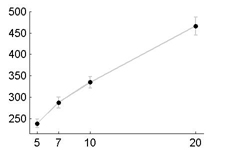The Basic usage of the plotting functions is lineplot(x,y). The Function plots the mean and standard error for every category in x. x can be either numerical or a string varaible (cell-array). y has to be numerical. Splitting data lineplot([D.feedback D.ampl],D.MT,... 'split',D.Group);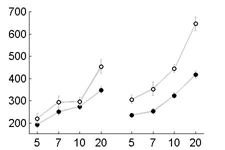We now introduced two x-variables varaibles (x has two columns). Lineplot first groups the data by feedback (no-feeback on the left, feedback on the right) and then by amplitude. The data is 'split' by the variable D.Group and seperate lines are drawn for each category. Subsetting data lineplot(D.ampl,D.MT,...'split',D.Group,...'subset',D.feedback==0);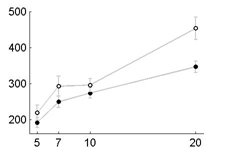We can exclude data from plotting by specifying a subset-variable. This time we only plot the no-feedback data. Plotting statistic other than mean lineplot(D.ampl,D.MT,...'split',D.Group,... 'plotfcn','(std(x)/2)',...'errorfcn',[]);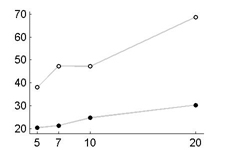Instead of plotting the mean of the data, we can also decide to plot any arbitrary function on the data, for example half the standard deviation. The same manipulation works for the length of the errorbars (for asymmetric error bars, see help). Basic formatting lineplot(D.ampl,D.MT,...'split',D.Group,... 'markersize',5,...'linewidth',2);By specifying the values for basic formatting properties, we can specify the format for all the lines/symbols in the graph (for full list see help lineplot). Specific formatting CAT.markertype={'v','^'};CAT.linecolor=... {[1 0 0],[0 0 1]}; CAT.markercolor=... {[1 0 0],[0 0 1]}; CAT.markerfill=... {[0 0 0],[0 0 0]}; lineplot([D.ampl],D.MT,...'split',D.Group,... 'CAT',CAT);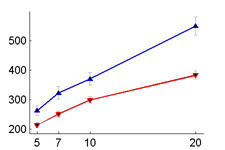If different styles have to be applied to different lines, specify fields in a CAT-structure, each with as many entries as you have categories. If fields are unspecified, the default (or global value) is used. Styles lineplot([D.ampl],D.MT,...'split',D.Group,... 'style_thickline');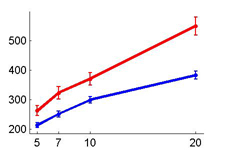Instead of having to define a style each time, you can use predetermined styles. They are easy to add to the code. Adding a legend lineplot([D.ampl],D.MT,...'split',D.Group,... 'style_thickline',...'leg','auto');For adding a legend, set option 'leg' to 'auto'. This uses the values of the split-varaible to determine the legend text. You can also specify the legend texts directly by setting 'leg' to a cell-array of strings.

Examples of graph types

 boxplot myboxplot(D.ampl,D.MT,...'split',D.Group,...'style_tukey',...'leg','auto');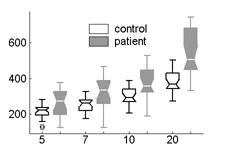Boxplots (introduced by Tukey) reveal the median and distribution of small samples. barplot barplot(D.Group,D.accuracy,...'split',D.ampl,...'style_bold',...'leg','auto');Barplots are good for categorical data with few classes. scatterplot scatterplot(D.MT,D.accuracy,...'split',D.Group);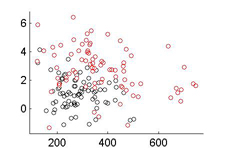Scatterplots show for seperate groups the relationship between two variables (see scattermatrix for the relation between more than two variables). histograms hist_double(x,y);Histograms show the frequency of observations, here overlaid for two random variables (see also hist_pivot). kernel estimates contour_kernel(x,y);A density estimate using kernel methods. Function kernel estimates are also possible. hist2d hist2d(x,y,'image');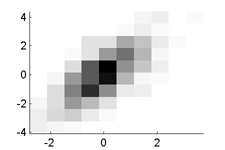The same data as a 2-d histogram. (see also contour_pivot).

List of functions

 1. Single plot-operations / Utility functions drawline draws vertical or horizontal reference lines drawpatch draws a patch of certain shape confidelps draws Confidence-ellipse over the x,y sample makexlabels Makes labels for the x-axis of Grouped graphs makeglabels Makes group labels for boxplots, etc. errorbars Adds errorbars to bargraphs 2. Standard plots from raw data, many formating options barplot Barplots from raw data lineplot Lineplots from raw data myboxplot Boxplots with formating options xyplot XY-plot with error bars in both directions scatterplot Scatterplot with formating/splitting options trialscatter Scatter of a time series of experimental trials with block boundaries traj_plot Plots trajectories in 2D traceplot Plotting of multiple time series with formating options scattermatrix Matrix of scatterplots to access dependency of variables plotshade Plots times series with +-SD as transparent underlay hist_double Overlays the histograms for two variables hist_pivot Makes M*N subplots with histograms, depending on categorial variabels histdistn_plot Overlays a histogram with a distribution function (Scaled) circhist Circular histogram (see circ toolbox) 3. Multivariate surface plots hist2d Two dimensional histogram presented as a image contour_pivot Makes a contour plot from a pivot-table contour_kernel Makes a contour plot from a kernel estimate (density or function) 4. Formating figure_scalesubplots Scales all subplots to the same axis-limits figure_scalexsubplots Scales all subplots to the same axis-limits figure_scaleysubplots Scales all subplots to the same axis-limits wysiwyg Present Figure on Screen as it is printedJörn Diedrichsen'sHomepageMatlabToolboxes